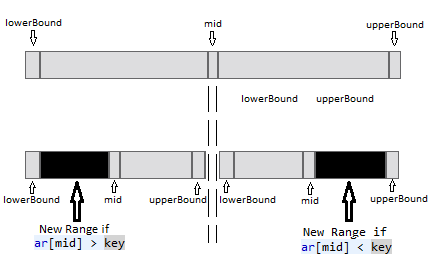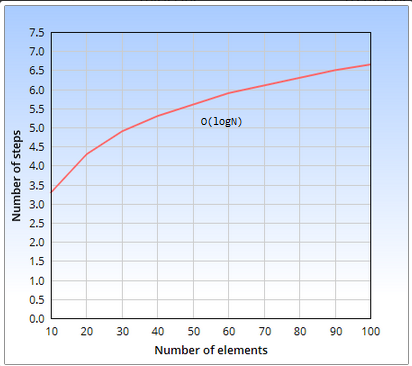### Binary Search (without Recursion) in java

We may also use simple way of searching i.e. Linear Search which is slower than Binary Search.

We’ll be using the BinarySearchArray class to encapsulate the array and its algorithms.
The most important method of this class is the binarySearch() method, which uses a binary search to locate a specified data item.
The binarySearch() method searches for a specified item by repeatedly dividing in half the
range of array elements to be considered.

The method looks like this:
/**
* Find element using Binary search
*/
public Integer binarySearch(int key) {
int low = 0;  //points to first occupied cell in array.
int up = index - 1; //points to last occupied cell in array.
int mid = 0;  //points to mid of array.
while (true) {
mid = (low + up) / 2;
if (low > up) {
return index;
} else if (ar[mid] == key) {
System.out.print("\nElement=" + key + " found at position="   + mid);
return mid;
} else if (ar[mid] > key) {
up = mid - 1;
} else {
low = mid + 1;
}
}
}

Logic explanation >

The method begins by setting lowerBound and upperBound variables to the first and
last occupied cells in the array.
We also define mid variable variable mid which points to middle position of array.
Setting these variables specifies the range where the item we’re looking for, key, can be found.

If mid points to the desired item, so we first check if this is true. If it is, we’ve found the item, so we return with mid(as it is position at which we have found element).
Each time through the loop we divide the range in half. Ultimately, the range will get so small that it can’t be divided any more. We check this in the next statement-
If lowerBound is greater than upperBound (Element has not been found and we return).

If mid is not pointing at the desired item, and the range is still big enough, we’ll divide the range in half. We compare the value at the current index, a[mid], which is in the middle of the range, with the value to be found i.e. key.

Now there can be 2cases>
Case1>
If key is larger, we know we should look in the upper half of the range.
Accordingly, we move lowerBound up to mid. Actually, we move it one cell beyond
mid because we’ve already checked mid itself at the beginning of the loop.
Case2>
If key is smaller than a[mid], we know we should look in the lower half of
the range. So we move upperBound down to one cell below mid.Full Program/SourceCode >

Complexity of Binary Search >

Best,average and worst case >

Best Case :   O(1) ; when key is middle element
Average Case : O(logN)
Worst Case :     O(logN)

>No. of comparisons : O(logN) in average/worst case
& 1 in best case(when key is middle element)

Explanation >
Complexity of Binary Search is O(logN), where N is number of elements

It doesn't half search time, that wouldn't make it log(n). It decreases it logarithmically.
If you had 128 entries in a table and had to search linearly for your value, it would probably take around 128 entries on average to find your value. That's log(n) or linear time.
With a binary search, you eliminate 1/2 the possible entries each iteration, such that at most it would only take 7 compares to find your value (log base 2 of 128 is 7 or 2 to the 7 power is 128.) This is the power of binary search.

Comparisons needed in Binary Search >

Searching 10 item in Binary search will take average of 4 searches.
Searching 100 item in Binary search will take average of 7 searches.
Searching 1000 item in Binary search will take average of 10 searches & so on….

 Range Comparisons Needed 10 4 100 7 1000 10 10,000 14 100,000 17 1,000,000 20 10,000,000 24 100,000,000 27 1,000,000,000 30

Complexity of Binary Search in graph >